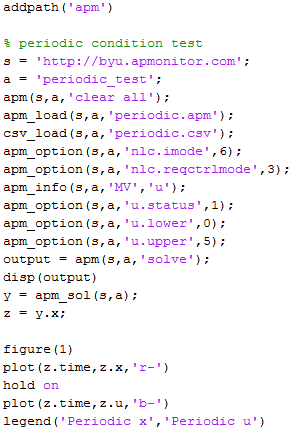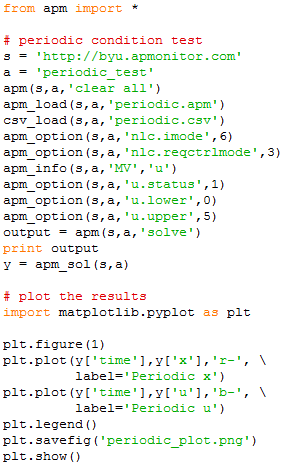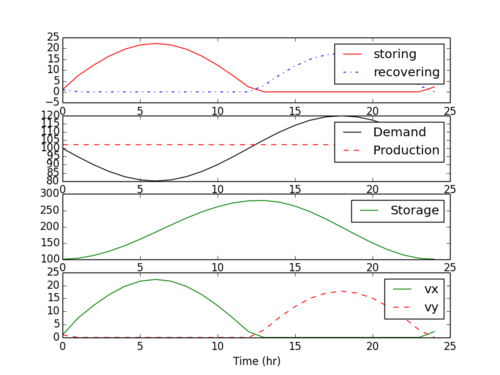## Apps.PeriodicBoundaryConditions History

March 30, 2021, at 10:19 PM by 10.35.117.248 -
Changed line 37 from:

$$0 \le u \ge 5$$

to:

$$0 \le u \le 5$$

March 10, 2021, at 03:21 PM by 10.35.117.248 -
Changed lines 218-219 from:
• Safdarnejad, S.M., Hedengren, J.D., Baxter, L.L.,

Plant-level dynamic optimization of Cryogenic Carbon Capture with conventional and renewable power sources, Applied Energy, Volume 149, 2015, Pages 354-366, ISSN 0306-2619, https://doi.org/10.1016/j.apenergy.2015.03.100 Article

to:
• Safdarnejad, S.M., Hedengren, J.D., Baxter, L.L., Plant-level dynamic optimization of Cryogenic Carbon Capture with conventional and renewable power sources, Applied Energy, Volume 149, 2015, Pages 354-366, ISSN 0306-2619, DOI: 10.1016/j.apenergy.2015.03.100. Article
March 10, 2021, at 03:21 PM by 10.35.117.248 -

#### Reference

• Safdarnejad, S.M., Hedengren, J.D., Baxter, L.L.,

Plant-level dynamic optimization of Cryogenic Carbon Capture with conventional and renewable power sources, Applied Energy, Volume 149, 2015, Pages 354-366, ISSN 0306-2619, https://doi.org/10.1016/j.apenergy.2015.03.100 Article

March 10, 2021, at 03:18 PM by 10.35.117.248 -
Changed lines 27-28 from:

The following example illustrates the use of the boundary condition. Scripts in MATLAB and Python are available below to recreate this solution along with the model equations in APMonitor. Both MATLAB and Python scripts produce equivalent results.

to:

An example illustrates the use of periodic boundary conditions.

$$\min_u \left(x-3\right)^2$$

$$\frac{dx}{dt}+x=cos(t)+u$$

$$x(0)=x(8)=1$$

$$u(0)=u(8)=1$$

$$0 \le u \ge 5$$Scripts in MATLAB and Python are available below to recreate this solution along with the model equations in APMonitor. Both MATLAB and Python scripts produce equivalent results.

March 10, 2021, at 03:02 PM by 10.35.117.248 -
Changed lines 53-54 from:Periodic Example Script in MATLAB/Python (periodic_example.zip)
to:Periodic Example Script in MATLAB/Python (zip file)
Changed line 198 from:Periodic Energy Storage in MATLAB/Python (periodic_energy_storage.zip)
to:Periodic Energy Storage in MATLAB/Python (zip file)
March 10, 2021, at 03:01 PM by 10.35.117.248 -
Changed lines 29-34 from:

APMonitor Model

(:toggle hide apmcode button show="Show APMonitor Model File":) (:div id=apmcode:)

to:

(:toggle hide gkcode button show="Show GEKKO Python Source":) (:div id=gkcode:)

from gekko import GEKKO import numpy as np m = GEKKO() m.time = np.linspace(0,8,81) t = m.Param(m.time) u = m.MV(1,lb=0,ub=5); u.STATUS=1 x = m.Var(1) m.periodic(u) m.periodic(x) m.Minimize((x-3)**2) m.Equation(x.dt()+x==m.cos(t)+u) m.options.IMODE = 6 m.solve()

import matplotlib.pyplot as plt plt.plot(m.time,u,m.time,x) plt.legend(['u','x']) plt.show() (:sourceend:) (:divend:)Periodic Example Script in MATLAB/Python (periodic_example.zip)

(:toggle hide apmcode button show="Show APMonitor Model File":) (:div id=apmcode:) (:source lang=python:)

Changed line 198 from:
to:Periodic Energy Storage in MATLAB/Python (periodic_energy_storage.zip)
March 10, 2021, at 02:49 PM by 10.35.117.248 -
Changed lines 7-8 from:

In the APMonitor software, boundary conditions are added for select variables with the use of a periodic object declaration.

to:

Boundary conditions are added for select variables with the use of a periodic object declaration.

APMonitor Model

Changed lines 17-19 from:

In Python Gekko, there is a periodic function.

to:

In Python Gekko, there is a periodic function to add the APMonitor periodic condition.

Python Gekko

March 10, 2021, at 02:48 PM by 10.35.117.248 -
Changed lines 7-21 from:

In the APMonitor software, boundary conditions are added for select variables with the use of a periodic object declaration. Linking this periodic object to a variable in the model enforces the periodic condition by adding an additional equation that the end point must be equal to the beginning point in the horizon.

to:

In the APMonitor software, boundary conditions are added for select variables with the use of a periodic object declaration.

(:source lang=python:) Objects

  q = periodic


End Objects (:sourceend:)

In Python Gekko, there is a periodic function.

(:source lang=python:) m.periodic(q) (:sourceend:)

Linking this periodic object to a variable in the model enforces the periodic condition by adding an additional equation that the end point must be equal to the beginning point in the horizon.

March 10, 2021, at 02:46 PM by 10.35.117.248 -
Changed lines 61-62 from:
to:

(:divend:)

(:toggle hide gekkocode button show="Show GEKKO Python Source":) (:div id=gekkocode:)

(:divend:)

March 10, 2021, at 02:45 PM by 10.35.117.248 -
Changed lines 15-17 from:

(:source lang=matlab:)

to:

(:toggle hide apmcode button show="Show APMonitor Model File":) (:div id=apmcode:) (:source lang=python:)

Changed lines 39-40 from:
  vx >= 0 !used for energy storage representation
vy >= 0	!used for energy recovery representation

to:
  vx >= 0  # slack variable for energy storage representation
vy >= 0  # slack variable for energy recovery representation

March 10, 2021, at 02:43 PM by 10.35.117.248 -
Changed lines 14-17 from:MATLAB Scriptto:

(:source lang=matlab:) Objects

  q = periodic


End Objects

Connections

  s = q.x


End Connections

Constants

  eps = 0.7


End Constants

Parameters

  d
p


End Parameters

Variables

  s >= 0 , = 100
stored
recovery
vx >= 0 !used for energy storage representation
vy >= 0	!used for energy recovery representation


End Variables

Equations

  minimize p
p + recovery/eps - stored >= d
p - d = vx- vy
stored = p-d + vy
recovery = d- p + vx
$s = stored - recovery/ eps stored * recovery <= 0  End Equations File *.plt  New Trend p s d  End File (:sourceend:) March 10, 2021, at 02:41 PM by 10.35.117.248 - Changed line 35 from:  http://apmonitor.com/wiki/index.php/Apps/PeriodicBoundaryConditions  to:  https://apmonitor.com/wiki/index.php/Apps/PeriodicBoundaryConditions  March 10, 2021, at 02:41 PM by 10.35.117.248 - Deleted lines 18-21: Python ScriptMarch 10, 2021, at 02:40 PM by 10.35.117.248 - Changed line 117 from:to:March 10, 2021, at 02:40 PM by 10.35.117.248 - Changed lines 29-31 from:to: (:source lang=python:) 1. !/usr/bin/env python3 2. -*- coding: utf-8 -*- """ Created on Mon Mar 8 21:34:49 2021 Gekko implementation of the simple energy storage model found here:  https://www.sciencedirect.com/science/article/abs/pii/S030626191500402X  Useful link:  http://apmonitor.com/wiki/index.php/Apps/PeriodicBoundaryConditions  @author: Nathaniel Gates, John Hedengren """ import numpy as np import matplotlib.pyplot as plt import matplotlib.ticker as mtick from gekko import GEKKO m = GEKKO(remote=False) t = np.linspace(0, 24, 24*3+1) m.time = t m.options.SOLVER = 1 m.options.IMODE = 6 m.options.NODES = 3 m.options.CV_TYPE = 1 m.options.MAX_ITER = 300 p = m.FV() # production p.STATUS = 1 s = m.Var(100, lb=0) # storage inventory store = m.SV() # store energy rate vy = m.SV(lb=0) # store slack variable recover = m.SV() # recover energy rate vx = m.SV(lb=0) # recover slack variable eps = 0.7 d = m.MV(-20*np.sin(np.pi*t/12)+100) m.periodic(s) m.Equations([p + recover/eps - store >= d,  p - d == vx - vy, store == p - d + vy, recover == d - p + vx, s.dt() == store - recover/eps, store * recover <= 0])  m.Minimize(p) m.solve(disp=True) 1. Visualize results fig, axes = plt.subplots(4, 1, sharex=True) ax = axes ax.plot(t, store, 'C3-', label='Store Rate') ax.plot(t, recover, 'C0-.', label='Recover Rate') ax = axes ax.plot(t, d, 'k-', label='Electricity Demand') ax.plot(t, p, 'C3--', label='Power Production') ax = axes ax.plot(t, s, 'C2-', label='Energy Inventory') ax = axes ax.plot(t, vx, 'C2-', label='$S_1$') ax.plot(t, vy, 'C3--', label='$S_2\$') ax.set_xlabel('Time (hr)')

for ax in axes:

    ax.legend(bbox_to_anchor=(1.01, 0.5),               loc='center left', frameon=False)
ax.grid()
ax.set_xlim(0, 24)
loc = mtick.MultipleLocator(base=6)
ax.xaxis.set_major_locator(loc)


plt.tight_layout() plt.show() (:sourceend:)May 28, 2014, at 10:49 PM by 107.188.175.164 -

#### Periodic Energy Storage

May 28, 2014, at 10:49 PM by 107.188.175.164 -May 28, 2014, at 10:48 PM by 107.188.175.164 -
Changed line 27 from:
to:
May 28, 2014, at 10:48 PM by 107.188.175.164 -
Changed lines 9-10 from:

The following example illustrates the use of the boundary condition. Scripts in MATLAB and Python are available below to recreate this solution.

to:

The following example illustrates the use of the boundary condition. Scripts in MATLAB and Python are available below to recreate this solution along with the model equations in APMonitor. Both MATLAB and Python scripts produce equivalent results.

Changed lines 13-16 from:
• MATLAB Script
to:

APMonitor ModelMATLAB Script

Changed line 19 from:
• Python Script
to:

Python ScriptA further example demonstrates a more complicated model for energy storage and retrieval. In this case, energy is stored during the first hours of the day when demand is lower. The power generation runs at a constant level while the energy storage is able to follow the cyclical demand. Energy storage is set to a periodic boundary condition to ensure that the beginning and end of the day have at least 100 units of stored energy. Scripts are available in both MATLAB and Python.

May 28, 2014, at 10:42 PM by 107.188.175.164 -
Changed lines 13-18 from:

(:table border=1 width=100%:) (:cell:) MATLAB (:cell:) Python (:cellnr:)(:cell:)(:tableend:)

to:
• MATLAB Script• Python ScriptMay 28, 2014, at 10:36 PM by 107.188.175.164 -
Changed lines 16-17 from:

(:cellnr:) (:cell:)to:

(:cellnr:)May 28, 2014, at 10:35 PM by 107.188.175.164 -
(:table border=1 width=100%:) (:cell:) MATLAB (:cell:) Python (:cellnr:) (:cell:)(:cell:)(:tableend:)